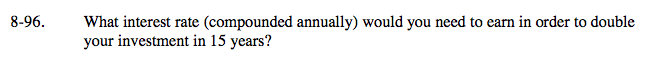### Home > A2C > Chapter 8 > Lesson 8.1.6 > Problem8-96

8-96.Compound interest is exponential.
Use the general equation y = abx where
a = initial investment,
b = multiplier,
x = number of years, and
y = total investment.

If you invest $100 initially, you will have$200 when it has doubled.
Input the values into the equation.

Divide both sides by 100 and take the 15th root of both sides

$\text{(or raise both sides to the }\frac{1}{15}\text{ power.)}$

Convert the multiplier to an interest rate, I.
Since this is an increasing exponential function, subtract 1 before converting to a percent.

200 = 100(b)15

b = 1.04729

I ≈ 0.04729 ≈ 4.73%